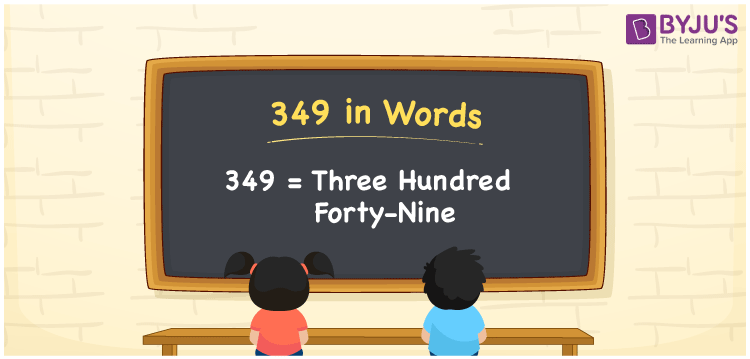# 349 in words

349 in words is written as Three Hundred and Forty Nine. 349 represents the count or value. The article on Counting Numbers can give you an idea about count or counting. The number 349 is a 3 digit number that is used in expressions related to money, days, distance, length, weight and so on. Let us consider an example for 349. “The bus route number Three Hundred and Forty Nine goes to my Grandparents place”.

 349 in words Three Hundred and Forty Nine Three Hundred and Forty Nine in Numbers 349

## 349 in English Words## How to Write 349 in Words?

We can convert 349 to words using a place value chart. The number 349 has 3 digits, so let’s make a chart that shows the place value up to 3 digits.

 Hundreds Tens Ones 3 4 9

Thus, we can write the expanded form as:

3 × Hundred + 4 × Ten + 9 × One

= 3 × 100 + 4 × 10 + 9 × 1

= 349

= Three Hundred and Forty Nine.

349 is the natural number that is succeeded by 348 and preceded by 350.

349 in words – Three Hundred and Forty Nine.

Is 349 an odd number? – Yes.

Is 349 an even number? – No.

Is 349 a perfect square number? – No.

Is 349 a perfect cube number? – No.

Is 349 a prime number? – Yes.

Is 349 a composite number? – No.

## Solved Example

1. Write the number 349 in expanded form

Solution: 3 × 100 + 4 × 10 + 9 × 1

We can write 349 = 300 + 40 + 9

= 3 × 100 + 4 × 10 + 9 × 1.

## Frequently Asked Questions on 349 in words

Q1

### How to write the number 349 in words?

349 in words is written as Three Hundred and Forty Nine.
Q2

### Is 349 divisible by 3?

No. 349 is not divisible by 3.
Q3

### Is 349 a prime number?

Yes. 349 is a prime number.